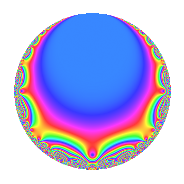# Properties

 Label 201.3.g.aLevel 201 Weight 3 Character orbit 201.g Analytic conductor 5.477 Analytic rank 0 Dimension 2 CM disc. -3 Inner twists 4

# Related objects

## Newspace parameters

 Level: $$N$$ = $$201 = 3 \cdot 67$$ Weight: $$k$$ = $$3$$ Character orbit: $$[\chi]$$ = 201.g (of order $$6$$ and degree $$2$$)

## Newform invariants

 Self dual: No Analytic conductor: $$5.47685331364$$ Analytic rank: $$0$$ Dimension: $$2$$ Coefficient field: $$\Q(\sqrt{-3})$$ Coefficient ring: $$\Z[a_1, \ldots, a_{13}]$$ Coefficient ring index: $$1$$ Sato-Tate group: $\mathrm{U}(1)[D_{6}]$

## $q$-expansion

Coefficients of the $$q$$-expansion are expressed in terms of a primitive root of unity $$\zeta_{6}$$. We also show the integral $$q$$-expansion of the trace form.

 $$f(q)$$ $$=$$ $$q -3 q^{3} + ( -4 + 4 \zeta_{6} ) q^{4} + ( -2 + 2 \zeta_{6} ) q^{7} + 9 q^{9} +O(q^{10})$$ $$q -3 q^{3} + ( -4 + 4 \zeta_{6} ) q^{4} + ( -2 + 2 \zeta_{6} ) q^{7} + 9 q^{9} + ( 12 - 12 \zeta_{6} ) q^{12} -23 \zeta_{6} q^{13} -16 \zeta_{6} q^{16} -26 \zeta_{6} q^{19} + ( 6 - 6 \zeta_{6} ) q^{21} + 25 q^{25} -27 q^{27} -8 \zeta_{6} q^{28} + ( 13 - 13 \zeta_{6} ) q^{31} + ( -36 + 36 \zeta_{6} ) q^{36} -26 \zeta_{6} q^{37} + 69 \zeta_{6} q^{39} -61 q^{43} + 48 \zeta_{6} q^{48} + 45 \zeta_{6} q^{49} + 92 q^{52} + 78 \zeta_{6} q^{57} -47 \zeta_{6} q^{61} + ( -18 + 18 \zeta_{6} ) q^{63} + 64 q^{64} + ( -77 + 45 \zeta_{6} ) q^{67} -143 \zeta_{6} q^{73} -75 q^{75} + 104 q^{76} + ( -131 + 131 \zeta_{6} ) q^{79} + 81 q^{81} + 24 \zeta_{6} q^{84} + 46 q^{91} + ( -39 + 39 \zeta_{6} ) q^{93} -167 \zeta_{6} q^{97} +O(q^{100})$$ $$\operatorname{Tr}(f)(q)$$ $$=$$ $$2q - 6q^{3} - 4q^{4} - 2q^{7} + 18q^{9} + O(q^{10})$$ $$2q - 6q^{3} - 4q^{4} - 2q^{7} + 18q^{9} + 12q^{12} - 23q^{13} - 16q^{16} - 26q^{19} + 6q^{21} + 50q^{25} - 54q^{27} - 8q^{28} + 13q^{31} - 36q^{36} - 26q^{37} + 69q^{39} - 122q^{43} + 48q^{48} + 45q^{49} + 184q^{52} + 78q^{57} - 47q^{61} - 18q^{63} + 128q^{64} - 109q^{67} - 143q^{73} - 150q^{75} + 208q^{76} - 131q^{79} + 162q^{81} + 24q^{84} + 92q^{91} - 39q^{93} - 167q^{97} + O(q^{100})$$

## Character Values

We give the values of $$\chi$$ on generators for $$\left(\mathbb{Z}/201\mathbb{Z}\right)^\times$$.

 $$n$$ $$68$$ $$136$$ $$\chi(n)$$ $$-1$$ $$-1 + \zeta_{6}$$

## Embeddings

For each embedding $$\iota_m$$ of the coefficient field, the values $$\iota_m(a_n)$$ are shown below.

For more information on an embedded modular form you can click on its label.

Label $$\iota_m(\nu)$$ $$a_{2}$$ $$a_{3}$$ $$a_{4}$$ $$a_{5}$$ $$a_{6}$$ $$a_{7}$$ $$a_{8}$$ $$a_{9}$$ $$a_{10}$$
29.1
 0.5 − 0.866025i 0.5 + 0.866025i
0 −3.00000 −2.00000 3.46410i 0 0 −1.00000 1.73205i 0 9.00000 0
104.1 0 −3.00000 −2.00000 + 3.46410i 0 0 −1.00000 + 1.73205i 0 9.00000 0
 $$n$$: e.g. 2-40 or 990-1000 Significant digits: Format: Complex embeddings Normalized embeddings Satake parameters Satake angles

## Inner twists

Char. orbit Parity Mult. Self Twist Proved
1.a Even 1 trivial yes
3.b Odd 1 CM by $$\Q(\sqrt{-3})$$ yes
67.c Even 1 yes
201.g Odd 1 yes

## Hecke kernels

This newform can be constructed as the kernel of the linear operator $$T_{2}$$ acting on $$S_{3}^{\mathrm{new}}(201, [\chi])$$.